The Australian Journal of Mathematical Analysis and Applications

 Home News Editors Volumes RGMIA Subscriptions Authors Contact

ISSN 1449-5910

Sorry death is imminent for file: ../public_html/searchroot/files/tex/v19n2/v19i2p4.tex
You searched for xu
Total of 239 results found in site

44: Paper Source PDF document

Paper's Title:

Comparison Results for Solutions of Time Scale Matrix Riccati Equations and Inequalities

Author(s):

R. Hilscher

Department of Mathematical Analysis, Faculty of Science,
Masaryk University, Jan
áčkovo nám. 2a,
CZ-60200, Brno, Czech Republic.
hilscher@math.muni.cz
URL: http://www.math.muni.cz/~hilscher/

Abstract:

In this paper we derive comparison results for Hermitian solutions of time scale matrix Riccati equations and Riccati inequalities. Such solutions arise from special conjoined bases (X,U) of the corresponding time scale symplectic system via the Riccati quotient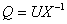. We also discuss properties of a unitary matrix solution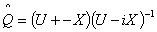of a certain associated Riccati equation.

12: Paper Source PDF document

Paper's Title:

Shape Diagrams for 2D Compact Sets - Part I: Analytic Convex Sets.

Author(s):

S. Rivollier, J. Debayle and J.-C. Pinoli

Ecole Nationale Supérieure des Mines de Saint-Etienne,

CIS - LPMG, UMR CNRS 5148, 158 cours Fauriel,

42023 Saint-Etienne Cedex 2, France.

Abstract:

Shape diagrams are representations in the Euclidean plane introduced to study 3-dimensional and 2-dimensional compact convex sets. Such a set is represented by a point within a shape diagram whose coordinates are morphometrical functionals defined as normalized ratios of geometrical functionals. Classically, the geometrical functionals are the area, the perimeter, the radii of the inscribed and circumscribed circles, and the minimum and maximum Feret diameters. They allow thirty-one shape diagrams to be built. Most of these shape diagrams can also been applied to more general compact sets than compact convex sets. Starting from these six classical geometrical functionals, a detailed comparative study has been performed in order to analyze the representation relevance and discrimination power of these thirty-one shape diagrams. The purpose of this paper is to present the first part of this study, by focusing on analytic compact convex sets. A set will be called analytic if its boundary is piecewise defined by explicit functions in such a way that the six geometrical functionals can be straightforwardly calculated. The second and third part of the comparative study are published in two following papers [19.20]. They are focused on analytic simply connected sets and convexity discrimination for analytic and discretized simply connected sets, respectively.

11: Paper Source PDF document

Paper's Title:

Iterative Approximation of Common Fixed Points of a Finite Family of Asymptotically Hemi-contractive Type Mappings

Author(s):

Jui-Chi Huang

Center for General Education,
Northern Taiwan Institute of Science and Technology,
Peito, Taipei,
Taiwan, 11202, R.O.C.
juichi@ntist.edu.tw

Abstract:

In this paper, we prove that the sequence of the modified Ishikawa-Xu,
Ishikawa-Liu, Mann-Xu and Mann-Liu iterative types of a finite family of
asymptotically hemi-contractive type mappings converges strongly to a common
fixed point of the family in a real p-uniformly convex Banach space with
p>1. Our results improve and extend some recent results.

11: Paper Source PDF document

Paper's Title:

Ostrowski Type Inequalities for Lebesgue Integral: a Survey of Recent Results

Author(s):

Sever S. Dragomir1,2

1Mathematics, School of Engineering & Science
Victoria University, PO Box 14428
Melbourne City, MC 8001,
Australia
E-mail: sever.dragomir@vu.edu.au

2DST-NRF Centre of Excellence in the Mathematical and Statistical Sciences,
School of Computer Science & Applied Mathematics,
University of the Witwatersrand,
Private Bag 3, Johannesburg 2050,
South Africa
URL: http://rgmia.org/dragomir

Abstract:

The main aim of this survey is to present recent results concerning Ostrowski type inequalities for the Lebesgue integral of various classes of complex and real-valued functions. The survey is intended for use by both researchers in various fields of Classical and Modern Analysis and Mathematical Inequalities and their Applications, domains which have grown exponentially in the last decade, as well as by postgraduate students and scientists applying inequalities in their specific areas.

11: Paper Source PDF document

Paper's Title:

An Integration Technique for Evaluating Quadratic Harmonic Sums

Author(s):

J. M. Campbell and K.-W. Chen

Department of Mathematics and Statistics,
York University, 4700 Keele St, Toronto,
ON M3J 1P3,
E-mail: jmaxwellcampbell@gmail.com

Department of Mathematics, University of Taipei,
Taipei 10048, Taiwan.
E-mail: kwchen@uTaipei.edu.tw
URL: https://math.utaipei.edu.tw/p/412-1082-22.php

Abstract:

The modified Abel lemma on summation by parts has been applied in many ways recently to determine closed-form evaluations for infinite series involving generalized harmonic numbers with an upper parameter of two. We build upon such results using an integration technique that we apply to ``convert'' a given evaluation for such a series into an evaluation for a corresponding series involving squared harmonic numbers.

8: Paper Source PDF document

Paper's Title:

C*-valued metric projection and Moore-Penrose inverse on Hilbert C*-modules

Author(s):

M. Eshaghi Gordji, H. Fathi and S.A.R. Hosseinioun

Department of Mathematics,
Semnan University, P.O. Box 35195-363, Semnan,
Iran.
Center of Excellence in Nonlinear Analysis and Applications (CENAA),
Semnan University,
Iran.

Department of Mathematics,
Shahid Beheshti University, Tehran,
Iran.
E-mail: Hedayat.fathi@yahoo.com

Department of Mathematical Sciences,
University of Arkansas, Fayetteville, Arkansas 72701,
USA.
E-mail: shossein@uark.net

Abstract:

Let t be a regular operator between Hilbert C*-modules and t be its Moore-Penrose inverse. We give some characterizations for t based on C*-valued metric projection. Moore-Penrose inverse of bounded operators and elements of a C*-algebra is studied as a special case.

8: Paper Source PDF document

Paper's Title:

MSplit Equality for Monotone Inclusion Problem and Fixed Point Problem in Real Banach Spaces

Author(s):

1,2Christian Chibueze Okeke, 3Abdumalik Usman Bello, 1Chinedu Izuchukwu, and 1Oluwatosin Temitope Mewomo

1School of Mathematics,
Statistics and Computer Science,
University of KwaZulu-Natal, Durban,
South Africa.
E-mail: okekec@ukzn.ac.za
E-mail: izuchukwuc@ukzn.ac.za
E-mail: mewomoo@ukzn.ac.za

2DST-NRF Center of Excellence in Mathematical and Statistical Sciences (CoE-Mass)
Johannesburg,
South Africa.

3Federal University,
Dutsin-Ma, Katsina State,
Nigeria.
E-mail: uabdulmalik@fudutsinma.edu.ng

Abstract:

In this paper a new iterative algorithm for approximating a common solution of split equality monotone inclusion problem and split equality fixed point problem is introduced. Using our algorithm, we state and prove a strong convergence theorem for approximating an element in the intersection of the set of solutions of a split equality monotone inclusion problem and the set of solutions of a split equality fixed point problem for right Bregman strongly nonexpansive mappings in the setting of p-uniformly convex Banach spaces which are also uniformly smooth. We also give some applications.

7: Paper Source PDF document

Paper's Title:

An Algorithm to Compute Gaussian-Type Quadrature Formulae
that Integrate Polynomials and Some Spline Functions Exactly

Author(s):

Allal Guessab

Laboratoire de Mathématiques Appliquées,
Université de Pau, 64000, Pau,
France.
allal.guessab@univ-pau.fr
URL: http://www.univ-pau.fr/~aguessab/

Abstract:

It is well-known that for sufficiently smooth integrands on an interval, numerical integration can be performed stably and efficiently via the classical (polynomial) Gauss quadrature formulae. However, for many other sets of integrands these quadrature formulae do not perform well. A very natural way of avoiding this problem is to include a wide class among arbitrary functions (not necessary polynomials) to be integrated exactly. The spline functions are natural candidates for such problems. In this paper, after studying Gaussian type quadrature formulae which are exact for spline functions and which contain boundary terms involving derivatives at both end points, we present a fast algorithm for computing their nodes and weights. It is also shown, taking advantage of the close connection with ordinary Gauss quadrature formula, that the latter are computed, via eigenvalues and eigenvectors of real symmetric tridiagonal matrices. Hence a new class of quadrature formulae can then be computed directly by standard software for ordinary Gauss quadrature formula. Comparative results with classical Gauss quadrature formulae are given to illustrate the numerical performance of the approach.

7: Paper Source PDF document

Paper's Title:

On Convergence Theorems of an Implicit Iterative Process with Errors for a Finite Family of Asymptotically quasi I-nonexpansive Mappings

Author(s):

Farrukh Mukhamedov and Mansoor Saburov

Department of Computational & Theoretical Sciences,
Faculty of Sciences, International Islamic University Malaysia,
P.O. Box, 141, 25710, Kuantan,
Malaysia

farrukh_m@iiu.edu.my

msaburov@gmail.com

http://www.iium.edu.my

Abstract:

In this paper we prove the weak and strong convergence of the implicit iterative process with errors to a common fixed point of a finite family {Tj}Ni=1 of asymptotically quasi Ij-nonexpansive mappings as well as a family of {Ij}Nj=1 of asymptotically quasi nonexpansive mappings in the framework of Banach spaces. The obtained results improve and generalize the corresponding results in the existing literature.

7: Paper Source PDF document

Paper's Title:

Cubic Alternating Harmonic Number Sums

Author(s):

Anthony Sofo

Victoria University,
College of Engineering and Science,
Melbourne City,
Australia.
E-mail: Anthony.Sofo@vu.edu.au

Abstract:

We develop new closed form representations of sums of cubic alternating harmonic numbers and reciprocal binomial coefficients. We also identify a new integral representation for the ζ (4)  constant.

7: Paper Source PDF document

Paper's Title:

Dynamical Analysis of HIV/AIDS Epidemic Model with Two Latent Stages, Vertical Transmission and Treatment

Author(s):

Nur Shofianah, Isnani Darti, Syaiful Anam

Mathematics Department,Faculty of Mathematics and Natural Sciences.
University of Brawijaya,
Jl. Veteran, Malang 65145,
Indonesia.
E-mail: nur_shofianah@ub.ac.id, isnanidarti@ub.ac.id, syaiful@ub.ac.id

Abstract:

We discuss about dynamical analysis of HIV/AIDS epidemic model with two latent stages, vertical transmission and treatment. In this model, the spreading of HIV occurs through both horizontal and vertical transmission. There is also treatment for individual who has been HIV infected. The latent stage is divided into slow and fast latent stage based on the immune condition which varies for each individual. Dynamical analysis result shows that the model has two equilibrium points: the disease-free equilibrium point and the endemic equilibrium point. The existence and global stability of equilibrium points depend on the basic reproduction number R0. When R0 <1, only the disease-free equilibrium point exists. If R0 >1, there are two equilibrium points, which are the disease-free equilibrium point and the endemic equilibrium point. Based on the result of stability analysis, the disease-free equilibrium point is globally asymptotically stable if R0 <1, while if R0 > 1 and p=q, the endemic equilibrium point will be globally asymptotically stable. In the end, we show some numerical simulations to support the analytical result.

7: Paper Source PDF document

Paper's Title:

Algorithms for Nonlinear Problems Involving Strictly Pseudocontractive Mappings

Author(s):

Mathew Olajiire Aibinu1, Surendra Colin Thakur2, Sibusiso Moyo3

1Institute for Systems Science & KZN E-Skill CoLab,
Durban University of Technology,
Durban 4000,
South Africa.

1DSI-NRF Centre of Excellence in Mathematical and Statistical Sciences (CoE-MaSS),
Johannesburg,
South Africa.
E-mail:
moaibinu@yahoo.com mathewa@dut.ac.za

2 KZN E-Skill CoLab,
Durban University of Technology,
Durban 4000,
South Africa.
E-mail:
thakur@dut.ac.za

3Institute for Systems Science & Office of the DVC Research, Innovation & Engagement Milena Court,
Durban University of Technology,
Durban 4000,
South Africa.
E-mail:
dvcrie@dut.ac.za

Abstract:

The puzzles in approximating a fixed point of nonlinear problems involving the class of strictly pseudocontractive mappings are conquered in this paper through viscosity implicit rules. Using generalized contraction mappings, a new viscosity iterative algorithm which is implicit in nature is proposed and analysed in Banach spaces for the class of strictly pseudocontractive mappings. The computations and analysis which are used in the proposed scheme are easy to follow and this gives rooms for a broad application of the scheme. It is obtained that the proposed iterative algorithm converges strongly to a fixed point of a μ-strictly pseudocontractive mapping which also solves a variational inequality problem. The result is also shown to hold for finite family of strictly pseudocontractive mappings. A numerical example is given to show the skillfulness of the proposed scheme and its implementation.

5: Paper Source PDF document

Paper's Title:

Iterative Approximation of Zeros of Accretive Type Maps, with Applications

Author(s):

Charles Ejike Chidume, Chinedu Godwin Ezea, and Emmanuel Ezzaka Otubo

African University of Science and Technology, Abuja,
Nigeria.
E-mail: cchidume@aust.edu.ng
E-mail: chinedu.ezea@gmail.com
E-mail: mrzzaka@yahoo.com

Department of Mathematics,
Nnamdi Azikiwe University,
Awka,
Nigeria
E-mail: chinedu.ezea@gmail.com

Ebonyi State University,
Abakaliki,
Nigeria
E-mail: mrzzaka@yahoo.com

Abstract:

Let E be a reflexive real Banach space with uniformly Gâteaux differentiable norm. Let J:E E* be the normalized duality map on E and let A:E* E be a map such that AJ is an accretive and uniformly continuous map. Suppose that (AJ)-1(0) in nonempty. Then, an iterative sequence is constructed and proved to converge strongly to some u* in (AJ)-1(0). Application of our theorem in the case that E is a real Hilbert space yields a sequence which converges strongly to a zero of A. Finally, non-trivial examples of maps A for which AJ is accretive are presented..

4: Paper Source PDF document

Paper's Title:

Essential Random Fixed Point Set of Random Operators

Author(s):

Ismat Beg

Centre for Advanced Studies in Mathematics,
Lahore University of Management Sciences (LUMS),
54792-Lahore, PAKISTAN. ibeg@lums.edu.pk
URL: http://web.lums.edu.pk/~ibeg

Abstract:

We obtain necessary and sufficient conditions for the existence of essential random fixed point of a random operator defined on a compact metric space. The structure of the set of essential random fixed points is also studied.

4: Paper Source PDF document

Paper's Title:

An Approximation of Jordan Decomposable Functions for a Lipschitz Function

Author(s):

Ibraheem Alolyan

Mathematics Department,
College of Science, King Saud University
Saudi Arabia
ialolyan05@yahoo.com

Abstract:

The well known Jordan decomposition theorem gives the useful characterization that any function of bounded variation can be written as the difference of two increasing functions. Functions which can be expressed in this way can be used to formulate an exclusion test for the recent Cellular Exclusion Algorithms for numerically computing all zero points or the global minima of functions in a given cellular domain [2,8,9]. In this paper we give an algorithm to approximate such increasing functions when only the values of the function of bounded variation can be computed. For this purpose, we are led to introduce the idea of ε-increasing functions. It is shown that for any Lipschitz continuous function, we can find two ε-increasing functions such that the Lipschitz function can be written as the difference of these functions.

4: Paper Source PDF document

Paper's Title:

Properties of Certain Multivalent Functions Involving Ruscheweyh Derivatives

Author(s):

N-Eng Xu and Ding-Gong Yang

Department of Mathematics,
Changshu Institute of Technology,
Changshu, Jiangsu 215500,
China

Abstract:

Let Ap(p∈ N) be the class of functions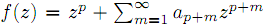which are analytic in the unit disk. By virtue of the Ruscheweyh derivatives we introduce the new subclasses Cp(n,α,β,λ,μ) of Ap. Subordination relations, inclusion relations, convolution properties and a sharp coefficient estimate are obtained. We also give a sufficient condition for a function to be in Cp(n,α,β,λ,μ)

4: Paper Source PDF document

Paper's Title:

Strong Convergence Theorem for a Common Fixed Point of an Infinite Family of J-nonexpansive Maps with Applications

Author(s):

Charlse Ejike Chidume, Otubo Emmanuel Ezzaka and Chinedu Godwin Ezea

African University of Science and Technology,
Abuja,
Nigeria.
E-mail: cchidume@aust.edu.ng

Ebonyi State University,
Abakaliki,
Nigeria.
E-mail: mrzzaka@yahoo.com

Nnamdi Azikiwe University,
Awka,
Nigeria.
E-mail: chinedu.ezea@gmail.com

Abstract:

Let E be a uniformly convex and uniformly smooth real Banach space with dual space E*. Let {Ti}i=1 be a family of J-nonexpansive maps, where, for each i,~Ti maps E to 2E*. A new class of maps, J-nonexpansive maps from E to E*, an analogue of nonexpansive self maps of E, is introduced. Assuming that the set of common J-fixed points of {Ti}i=1 is nonempty, an iterative scheme is constructed and proved to converge strongly to a point x* in n=1FJTi. This result is then applied, in the case that E is a real Hilbert space to obtain a strong convergence theorem for approximation of a common fixed point for an infinite family of nonexpansive maps, assuming existences. The theorem obtained is compared with some important results in the literature. Finally, the technique of proof is also of independent interest.

4: Paper Source PDF document

Paper's Title:

A New Method with Regularization for Solving Split Variational Inequality Problems in Real Hilbert Spaces

Author(s):

Francis Akutsah1 and Ojen Kumar Narain2

1School of Mathematics,
Statistics and Computer Science,
University of KwaZulu-Natal, Durban,
South Africa.
E-mail: 216040405@stu.ukzn.ac.za, akutsah@gmail.com

2School of Mathematics,
Statistics and Computer Science,
University of KwaZulu-Natal, Durban,
South Africa.
E-mail: naraino@ukzn.ac.za

Abstract:

In this paper, we introduce a new inertial extrapolation method with regularization for approximating solutions of split variational inequality problems in the frame work of real Hilbert spaces. We prove that the proposed method converges strongly to a minimum-norm solution of the problem without using the conventional two cases approach. In addition, we present some numerical experiments to show the efficiency and applicability of the proposed method. The results obtained in this paper extend, generalize and improve several results in this direction.

3: Paper Source PDF document

Paper's Title:

Inequalities Relating to the Gamma Function

Author(s):

Chao-Ping Chen and Feng Qi

Department of Applied Mathematics and Informatics, Research Institute of Applied Mathematics,
Henan Polytechnic University, Jiaozuo City, Henan 454000, China

chenchaoping@hpu.edu.cn

Department of Applied Mathematics and Informatics, Research Institute of Applied Mathematics,
Henan Polytechnic University, Jiaozuo City, Henan 454000, China

qifeng@hpu.edu.cn, fengqi618@member.ams.org

U
rl: http://rgmia.vu.edu.au/qi.html, http://dami.hpu.edu.cn/qifeng.html

Abstract:

For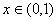, we have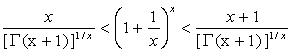.

For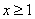,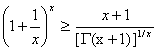,

And equality occurs for x=1.

3: Paper Source PDF document

Paper's Title:

Fixed Point Theorems for a Finite Family of Asymptotically Nonexpansive Mappings

Author(s):

E. Prempeh

Department of Mathematics,
Kwame Nkrumah University of Science and Technology,
Kumasi, Ghana
edward_prempeh2000@yahoo.com

Abstract:

Let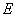be a real reflexive Banach space with a uniformly Gâteaux differentiable norm,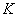be a nonempty bounded closed convex subset of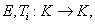i=1,2,...,r be a finite family of asymptotically nonexpansive mappings such that for each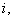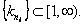Let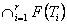be a nonempty set of common fixed points of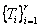and define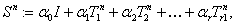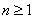. Let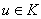be fixed and let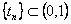be such that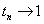as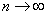. We can prove that the sequence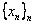satisfying the relation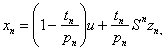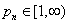associated with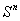, converges strongly to a fixed point of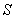providedpossesses uniform normal structure. Furthermore we prove that the iterative process: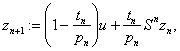, converges strongly to a fixed point of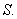3: Paper Source PDF document

Paper's Title:

Positive Solutions for Systems of Three-point Nonlinear Boundary Value Problems

Author(s):

J. Henderson and S. K. Ntouyas

Department of Mathematics, Baylor University
Waco, Texas
76798-7328 USA.
Johnny_Henderson@baylor.edu
URL: http://www3.baylor.edu/~Johnny_Henderson

Department of Mathematics, University of Ioannina
451 10 Ioannina,
Greece.
sntouyas@cc.uoi.gr
URL: http://www.math.uoi.gr/~sntouyas

Abstract:

Values of λ are determined for which there exist positive solutions of the system of three-point boundary value problems, u''(t)+ λa(t)f(v(t))=0, v''(t)+λb(t)g(u(t))=0, for 0 < t <1, and satisfying, u(0) = 0, u(1)=α u(η), v(0) = 0, v(1)=α v(η). A Guo-Krasnosel'skii fixed point theorem is applied.

3: Paper Source PDF document

Paper's Title:

On a Method of Proving the Hyers-Ulam Stability of Functional Equations on Restricted Domains

Author(s):

Janusz Brzdęk

Department of Mathematics
Pedagogical University Podchor
ąźych 2,
30-084 Krak
ów,
Poland
jbrzdek@ap.krakow.pl

Abstract:

We show that generalizations of some (classical) results on the Hyers-Ulam stability of functional equations, in several variables, can be very easily derived from a simple result on stability of a functional equation in single variable

3: Paper Source PDF document

Paper's Title:

On a Class of Meromorphic Functions of Janowski Type Related with a Convolution Operator

Author(s):

Abdul Rahman S. Juma, Husamaldin I. Dhayea

Department of Mathematics,
Iraq.
E-mail: dr_juma@hotmail.com

Department of Mathematics,
Tikrit University, Tikrit,
Iraq.

Abstract:

In this paper, we have introduced and studied new operator \$Qkλ,m,γ by the Hadamard product (or convolution) of two linear operators Dkλ and Im,γ, then using this operator to study and investigate a new subclass of meromorphic functions of Janowski type, giving the coefficient bounds, a sufficient condition for a function to belong to the considered class and also a convolution property. The results presented provide generalizations of results given in earlier works.

3: Paper Source PDF document

Paper's Title:

New Implicit Kirk-Type Schemes for General Class of Quasi-Contractive Operators in Generalized Convex Metric Spaces

Author(s):

K. Rauf, O. T. Wahab and A. Ali

Department of Mathematics,
University of Ilorin, Ilorin,
Nigeria.
E-mail: krauf@unilorin.edu.ng

Department of Statistics and Mathematical Sciences,
Kwara State University, Malete,
Nigeria.

Department of Mathematics,
Mirpur University of Science and Technology, Mirpur,
Pakistan.

Abstract:

In this paper, we introduce some new implicit Kirk-type iterative schemes in generalized convex metric spaces in order to approximate fixed points for general class of quasi-contractive type operators. The strong convergence, T-stability, equivalency, data dependence and convergence rate of these results were explored. The iterative schemes are faster and better, in term of speed of convergence, than their corresponding results in the literature. These results also improve and generalize several existing iterative schemes in the literature and they provide analogues of the corresponding results of other spaces, namely: normed spaces, CAT(0) spaces and so on.

3: Paper Source PDF document

Paper's Title:

Coefficient Estimates for Certain Subclasses of Bi-univalent Sakaguchi Type Functions by using Faber Polynomial

Author(s):

P. Murugabharathi, B. Srutha Keerthi

Mathematics Division,
Chennai - 600 127, India.
E-mail: bharathi.muhi@gmail.com
E-mail: sruthilaya06@yahoo.co.in

Abstract:

In this work, considering a general subclass of bi-univalent Sakaguchi type functions, we determine estimates for the general Taylor-Maclaurin coefficients of the functions in these classes. For this purpose, we use the Faber polynomial expansions. In certain cases, our estimates improve some of those existing coefficient bounds.

3: Paper Source PDF document

Paper's Title:

On Closed Range C*-modular Operators

Author(s):

Department of Mathematics,
Faculty of Mathematics and Statistics Sciences,
University of Birjand, Birjand,
Iran.

Abstract:

In this paper, for the class of the modular operators on Hilbert C*-modules, we give the conditions to closedness of their ranges. Also, the equivalence conditions for the closedness of the range of the modular projections on Hilbert C*-modules are discussed. Moreover, the mixed reverse order law for the Moore-Penrose invertible modular operators are given.

3: Paper Source PDF document

Paper's Title:

An Analytical Solution of Perturbed Fisher's Equation Using Homotopy Perturbation Method (HPM), Regular Perturbation Method (RPM) and Adomian Decomposition Method (ADM)

Author(s):

Moussa Bagayogo, Youssouf Minoungou, Youssouf Pare

Departement de Mathematique,
Universite Ouaga I Pr Joseph Ki-Zerbo,
Burkina Faso.
E-mail: moussabagayogo94@gmail.com, m.youl@yahoo.fr, pareyoussouf@yahoo.fr.

Abstract:

In this paper, Homotopy Perturbation Method (HPM), Regular Pertubation Method (RPM) and Adomian decomposition Method (ADM) are applied to Fisher equation. Then, the solution yielding the given initial conditions is gained. Finally, the solutions obtained by each method are compared

3: Paper Source PDF document

Paper's Title:

A Generalization of Ostrowski's Inequality for Functions of Bounded Variation via a Parameter

Author(s):

Seth Kermausuor

Department of Mathematics and Computer Science,
Alabama State University,
Montgomery, AL 36101,
USA.
E-mail: skermausour@alasu.edu

Abstract:

In this paper, we provide a generalization of the Ostrowski's inequality for functions of bounded variation for k points via a parameter λ∈[0,1]. As a by product, we consider some particular cases to obtained some interesting inequalities in these directions. Our results generalizes some of the results by Dragomir in [S. S. DRAGOMIR, The Ostrowski inequality for mappings of bounded variation, Bull. Austral. Math. Soc., 60 (1999), pp. 495--508.]

3: Paper Source PDF document

Paper's Title:

A Low Order Least-Squares Nonconforming Finite Element Method for Steady Magnetohydrodynamic Equations

Author(s):

Z. Yu, D. Shi and H. Zhu

College of Science,
Zhongyuan University of Technology,
Zhengzhou 450007,
China.
E-mail: 5772@zut.edu.cn

School of Mathematics and Statistics,
Zhengzhou University,
Zhengzhou 450001,
China.
E-mail: shi_dy@126.com

Mathematics Department,
University of Southern Mississippi,
Hattiesburg MS, 39406,
U.S.A
E-mail: huiqing.zhu@usm.edu

Abstract:

A low order least-squares nonconforming finite element (NFE) method is proposed for magnetohydrodynamic equations with EQ1rot element and zero-order Raviart-Thomas element. Based on the above element's typical interpolations properties, the existence and uniqueness of the approximate solutions are proved and the optimal order error estimates for the corresponding variables are derived.

3: Paper Source PDF document

Paper's Title:

On the Polyconvolution of Hartley Integral Transforms H1, H2, H1 and Integral Equations

Author(s):

Nguyen Minh Khoa and Dau Xuan Luong

Department of Mathematics,
Electric Power University,
Ha Noi, and Faculty of Fundamental Science,
Ha Long University, Quang Ninh,
Viet Nam.
E-mail: khoanm@epu.edu.vn, dauxuanluong@gmail.com

Abstract:

In this paper, we construct and study a new polyconvolution * (f,g,h)(x) of functions f, g, h. We will show that the polyconvolution satisfy the following factorization equality

H1[*(f,g,h)](y)=(H2f)(y)(H1g)(y)(H1h)(y), y R

We prove the existence of this polyconvolution in the space L(R). As examples, applications to solve an integral equation of polyconvolution type and two systems of integral equations of polyconvolution type are presented.

3: Paper Source PDF document

Paper's Title:

Optimal Control Analysis of HIV/AIDS Epidemic Model with an Antiretroviral Treatment

Author(s):

U. Habibah and R. A. Sari

Mathematics Department and Reseach Group of Biomathematics,
Faculty of Mathematics and Natural Science,
Brawijaya University, Jl. Veteran Malang 65145,
Indonesia.
E-mail: ummu_habibah@ub.ac.id

Abstract:

A mathematical model of HIV/AIDS is governed by a system of ordinary differential equations in the presence of an antiretroviral treatment (ARV). The theory of optimal control is applied to an epidemic model of HIV/AIDS which an ARV is used as a control strategy in order to prevent the spread of HIV/AIDS. The optimality system is derived by applying the Pontryagin's Minimum Principle. We analyze the boundedness and positivity of solutions, and an existence of the optimal control. Numerical simulations are conducted to obtain numerical solution of the optimally system.

2: Paper Source PDF document

Paper's Title:

Inclusion Properties of a Certain Subclass of Strongly Close-To-Convex Functions

Author(s):

S. M. Khairnar and M. More

Department of Mathematics,
Alandi -412 105, Pune, Maharashtra,
INDIA.

smkhairnar2007@gmail.com, meenamores@gmail.com.

Abstract:

The purpose of this paper is to derive some inclusion and argument properties of a new subclass of strongly close-to-convex functions in the open unit disc. We have considered an integral operator defined by convolution involving hypergeometric function in the subclass definition. The subclass also extends to the class of α-spirallike functions of complex order.

2: Paper Source PDF document

Paper's Title:

Some Distortion and Other Properties Associated with a Family of the n-Fold Symmetric Koebe Type Functions

Author(s):

H. M. Srivastava, N. Tuneski and E. Georgieva-Celakoska

Department of Mathematics and Statistics, University of Victoria,
Victoria, British Columbia V8W 3R4,

harimsri@math.uvic.ca

Faculty of Mechanical Engineering, St.
Cyril and Methodius University,
Karpo'v s II b.b., MK-1000 Skopje,
Republic of Macedonia

Abstract:

In a recent work by Kamali and Srivastava , a certain family of the n-fold symmetric Koebe type functions was introduced and studied systematically. In an earlier investigation, Eguchi and Owa  had considered its special case when n=1 (see also ). Here, in our present sequel to these earlier works, this general family of the n-fold symmetric Koebe type functions is studied further and several distortion theorems and such other properties as the radii of spirallikeness, the radii of starlikeness and the radii of convexity, which are associated with this family of the n-fold symmetric Koebe type functions, are obtained. We also provide certain criteria that embed this family of the n-fold symmetric Koebe type functions in a function class Gλ which was introduced and studied earlier by Silverman .

2: Paper Source PDF document

Paper's Title:

Generalized Weighted Trapezoid and Grüss Type Inequalities on Time Scales

Author(s):

Eze Raymond Nwaeze

Department of Mathematics,
Tuskegee University,
Tuskegee, AL 36088,
USA.
E-mail: enwaeze@mytu.tuskegee.edu

Abstract:

In this work, we obtain some new generalized weighted trapezoid and Grüss type inequalities on time scales for parameter functions. Our results give a broader generalization of the results due to Pachpatte in . In addition, the continuous and discrete cases are also considered from which, other results are obtained.

2: Paper Source PDF document

Paper's Title:

Some New Mappings Related to Weighted Mean Inequalities

Author(s):

Xiu-Fen Ma

College of Mathematical and Computer,
No.9 of Xuefu Road, Hechuan District 401520,
Chongqing City,
The People's Republic of China.
E-mail: maxiufen86@163.com

Abstract:

In this paper, we define four mappings related to weighted mean inequalities, investigate their properties, and obtain some new refinements of weighted mean inequalities.

2: Paper Source PDF document

Paper's Title:

Applications of Von Neumann Algebras to Rigidity Problems of (2-Step) Riemannian (Nil-)Manifolds

Author(s):

DFouman Faculty of Engineering,
College of Engineering, University of Tehran,
Iran.

Department of Mathematical Sciences,
Sharif University of Technology,
Iran
E-mail: fanai@sharif.edu

Abstract:

In this paper, basic notions of von Neumann algebra and its direct analogues in the realm of groupoids and measure spaces have been considered. By recovering the action of a locally compact Lie group from a crossed product of a von Neumann algebra, other proof of one of a geometric propositions of O'Neil and an extension of it has been proposed. Also, using the advanced exploration of nilmanifolds in measure spaces and their corresponding automorphisms (Lie algebraic derivations) a different proof of an analytic theorem of Gordon and Mao has been attained. These two propositions are of the most important ones for rigidity problems of Riemannian manifolds especially 2-step nilmanifolds.

2: Paper Source PDF document

Paper's Title:

Fractional exp(-φ(ξ))- Expansion Method and its Application to Space--Time Nonlinear Fractional Equations

Author(s):

A. A. Moussa and L. A. Alhakim

Department of Management Information System and Production Management,
College of Business and Economics, Qassim University,
P.O. BOX 6666, Buraidah: 51452,
Saudi Arabia.
E-mail: Alaamath81@gmail.com

Department of Management Information System and Production Management,
College of Business and Economics, Qassim University,
P.O. BOX 6666, Buraidah: 51452,
Saudi Arabia.
E-mail: Lama2736@gmail.com

Abstract:

In this paper, we mainly suggest a new method that depends on the fractional derivative proposed by Katugampola for solving nonlinear fractional partial differential equations. Using this method, we obtained numerous useful and surprising solutions for the space--time fractional nonlinear Whitham--Broer--Kaup equations and space--time fractional generalized nonlinear Hirota--Satsuma coupled KdV equations. The solutions obtained varied between hyperbolic, trigonometric, and rational functions, and we hope those interested in the real-life applications of the previous two equations will find this approach useful.

2: Paper Source PDF document

Paper's Title:

Author(s):

F. U. Ogbuisi

School of Mathematics, Statistics and Computer Science,
University of KwaZulu-Natal, Durban,
South Africa.

Department of Mathematics,
University of Nigeria, Nsukka,
Nigeria.
E-mail: ferdinard.ogbuisi@unn.edu.ng fudochukwu@yahoo.com

Abstract:

In this paper, we propose and analyze a type of subgradient extragradient algorithm for the approximation of a solution of variational inequality problem which is also a common fixed point of an infinite family of relatively nonexpansive mappings in 2-uniformly convex Banach spaces which are uniformly smooth. By using the generalized projection operator, we prove a strong convergence theorem which does not require the prior knowledge of the Lipschitz constant of cost operator. We further applied our result to constrained convex minimization problem, convex feasibility problem and infinite family of equilibrium problems. Our results improve and complement related results in 2-uniformly convex and uniformly smooth Banach spaces and Hilbert spaces.

2: Paper Source PDF document

Paper's Title:

Bounds for the Extremal Eigenvalues of Positive Definite Matrices

Author(s):

Shivani Singh and Pravin Singh

Unisa, Department of Decision Sciences,
PO Box 392,
Pretoria,
0003,
South Africa.
E-mail: singhs2@unisa.ac.za

University of KwaZulu-Natal,
School of Mathematics Statistics and Computer Sciences
Private Bag X54001,
Durban,
4000,
South Africa.
E-mail: singhprook@gmail.com

Abstract:

We use a projection to achieve bounds for a vector function of the eigenvalues of a positive definite matrix. For various choices of the monotonic function we are able to obtain bounds for the extremal eigenvalues in terms of the traces of the matrix and its powers. These bounds are relatively simple to compute.

1: Paper Source PDF document

Paper's Title:

New Reverses of Schwarz, Triangle and Bessel Inequalities in Inner Product Spaces

Author(s):

S. S. Dragomir

School of Computer Science and Mathematics, Victoria University of Technology, PO BOX
14428, MCMC 8001, VICTORIA, AUSTRALIA.

sever.dragomir@vu.edu.au
URL
: http://rgmia.vu.edu.au/SSDragomirWeb.html

Abstract:

New reverses of the Schwarz, triangle and Bessel inequalities in inner product spaces are pointed out. These results complement the recent ones obtained by the author in the earlier paper . Further, they are employed to establish new Grüss type inequalities. Finally, some natural integral inequalities are stated as well.

1: Paper Source PDF document

Paper's Title:

An alternative proof of monotonicity for the extended mean values

Author(s):

Chao-Ping Chen and Feng Qi

Department of Applied Mathematics and Informatics, Research Institute of Applied Mathematics,
Henan Polytechnic University, Jiaozuo City, Henan 454000, China

chenchaoping@hpu.edu.cn

Department of Applied Mathematics and Informatics, Research Institute of Applied Mathematics,
Henan Polytechnic University, Jiaozuo City, Henan 454000, China

qifeng@hpu.edu.cn, fengqi618@member.ams.org
Url
: http://rgmia.vu.edu.au/qi.html, http://dami.hpu.edu.cn/qifeng.html

Abstract:

An alternative proof of monotonicity for the extended mean values is given.

1: Paper Source PDF document

Paper's Title:

A Wallis Type Inequality and a Double Inequality for Probability Integral

Author(s):

Jian Cao, Da-Wei Niu and Feng Qi

School of Mathematics and Informatics,
Henan Polytechnic University, Jiaozuo City,
Henan Province, 454010,
China
21caojian@163.com

School of Mathematics and Informatics,
Henan Polytechnic University, Jiaozuo City,
Henan Province, 454010,
China
nnddww@tom.com

Research Institute of Mathematical Inequality Theory,
Henan Polytechnic University, Jiaozuo City,
Henan Province, 454010,
China
qifeng@hpu.edu.cn
fengqi618@member.ams.org
qifeng618@hotmail.com
qifeng618@msn.com
qifeng618@qq.com
URL: http://rgmia.vu.edu.au/qi.html

Abstract:

In this short note, a Wallis type inequality with the best upper and lower bounds is established. As an application, a double inequality for the probability integral is found.

1: Paper Source PDF document

Paper's Title:

A Different Proof for the non-Existence of Hilbert-Schmidt Hankel Operators with Anti-Holomorphic Symbols on the Bergman Space

Author(s):

Georg Schneider

Universität Wien, Brünnerstr. 72 A-1210 Wien,
Austria.
georg.schneider@univie.ac.at
URL: http://www.univie.ac.at/bwl/cont/mitarbeiter/schneider.htm

Abstract:

We show that there are no (non-trivial) Hilbert-Schmidt Hankel operators with anti-holomorphic symbols on the Bergman space of the unit-ball B2(Bl) for l≥2. The result dates back to . However, we give a different proof. The methodology can be easily applied to other more general settings. Especially, as indicated in the section containing generalizations, the new methodology allows to prove some robustness results for existing ones.

1: Paper Source PDF document

Paper's Title:

The Invariant Subspace Problem for Linear Relations on Hilbert Spaces

Author(s):

Daniel Grixti-Cheng

Department of Mathematics and Statistics,
The University of Melbourne,
Melbourne, VIC, 3010
Australia.
D.Grixti@ms.unimelb.edu.au

Abstract:

We consider the invariant subspace problem for linear relations on Hilbert spaces with the aim of promoting interest in the problem as viewed from the theory of linear relations. We present an equivalence between the single valued and multivalued invariant subspace problems and give some new theorems pertaining to the invariant subspace problem for linear relations on a Hilbert space.

1: Paper Source PDF document

Paper's Title:

Real Interpolation Methods and Quasilogarithmic Operators

Author(s):

Ming Fan

School of Industrial Technology and Management,
Dalarna University, 781 88 Borlänge, Sweden

fmi@du.se
URL: http://users.du.se/~fmi

Abstract:

The purpose of this paper is to deal with nonlinear quasilogarithmic operators, which possesses the uniformly bounded commutator property on various interpolation spaces in the sense of Brudnyi-Krugljak associated with the quasi-power parameter spaces. The duality, and the domain and range spaces of these operators are under consideration. Some known inequalities for the Lebesgue integration spaces and the trace classes are carried over to the non-commutative symmetric spaces of measurable operators affiliated with a semi-finite von Neumann algebra.

1: Paper Source PDF document

Paper's Title:

On Generalization of Hardy-type Inequalities

Author(s):

K. Rauf, S. Ponnusamy and J. O. Omolehin

Department of Mathematics,
University of Ilorin, Ilorin,
Nigeria
krauf@unilorin.edu.ng

Department of Mathematics,
Chennai- 600 036,
India
samy@iitm.ac.in

Department of Mathematics,
University of Ilorin, Ilorin,
Nigeria
omolehin_joseph@yahoo.com

Abstract:

This paper is devoted to some new generalization of Hardy-type integral inequalities and the reversed forms. The study is to determine conditions on which the generalized inequalities hold using some known hypothesis. Improvement of some inequalities are also presented.

1: Paper Source PDF document

Paper's Title:

Schwarz Method for Variational Inequalities Related to Ergodic Control Problems

Author(s):

Department of Mathematics, Badji Mokhtar University, Annaba 23000,
P.O.Box. 12, Annaba 23000, Algeria

signor_2000@yahoo.fr

Mcherihalima@yahoo.fr

Abstract:

In this paper, we study variational inequalities related to ergodic control problems studied by M. Boulbrachčne and H. Sissaoui , where the "discount factor" (i.e., the zero order term) is set to 0, we use an overlapping Schwarz method on nomatching grid which consists in decomposing the domain in two subdomains. For α ∈ ]0.1[ we provide the discretization on each subdomain converges in L -norm.

1: Paper Source PDF document

Paper's Title:

New Refinements of Hölder's Inequality

Author(s):

Xiu-Fen Ma

College of Mathematical and Computer,
No.9 of Xuefu Road, Hechuan District 401520,
Chongqing City,
The People's Republic of China.
E-mail: maxiufen86@163.com

Abstract:

In this paper, we define two mappings, investigate their properties, obtain some new refinements of Hölder's inequality.

1: Paper Source PDF document

Paper's Title:

Existence of Positive Solutions for Nonlinear Fractional Differential Equations with Multi-point Boundary Conditions

Author(s):

Khenchela University, Department of Mathematics,
Khenchela, 40000,
Algeria.

Abstract:

This paper is devoted to the existence results of positive solutions for a nonlinear fractional differential equations with multi-point boundary conditions. By means of the Schauder fixed point theorem, some results on the existence are obtained.

1: Paper Source PDF document

Paper's Title:

Convergence and Stability Results for New Three Step Iteration Process in Modular Spaces

Author(s):

Naresh Kumar and Renu Chugh

Department of Mathematics,
M.D. University,
Rohtak-124001, Haryana,
India.
E-mail: nks280@gmail.com
E-mail: chugh.r1@gmail.com

Abstract:

The aim of this paper is to introduce a new iteration process (5) for ρ-contraction mappings in Modular spaces. We obtain some analytical proof for convergence and stability of our iteration process (5). We show that our iteration process (5) gives faster convergence results than the leading AK iteration process (4) for contraction mappings. Moreover, a numerical example (using the Matlab Software) is presented to compare the rate of convergence for existing iteration processes with our new iteration process (5).

1: Paper Source PDF document

Paper's Title:

Inequalities for Discrete F-Divergence Measures: A Survey of Recent Results

Author(s):

Sever S. Dragomir1,2

1Mathematics, School of Engineering & Science
Victoria University, PO Box 14428
Melbourne City, MC 8001,
Australia
E-mail: sever.dragomir@vu.edu.au

2DST-NRF Centre of Excellence in the Mathematical and Statistical Sciences,
School of Computer Science & Applied Mathematics,
University of the Witwatersrand,
Private Bag 3, Johannesburg 2050,
South Africa
URL: http://rgmia.org/dragomir

Abstract:

In this paper we survey some recent results obtained by the author in providing various bounds for the celebrated f-divergence measure for various classes of functions f. Several techniques including inequalities of Jensen and Slater types for convex functions are employed. Bounds in terms of Kullback-Leibler Distance, Hellinger Discrimination and Varation distance are provided. Approximations of the f-divergence measure by the use of the celebrated Ostrowski and Trapezoid inequalities are obtained. More accurate approximation formulae that make use of Taylor's expansion with integral remainder are also surveyed. A comprehensive list of recent papers by several authors related this important concept in information theory is also included as an appendix to the main text.

1: Paper Source PDF document

Paper's Title:

Iterative Algorithm for Split Generalized Mixed Equilibrium Problem Involving Relaxed Monotone Mappings in Real Hilbert Spaces

Author(s):

1U.A. Osisiogu, F.L. Adum, and 2C. Izuchukwu

1Department of Mathematics and Computer Science,
Ebonyi State University, Abakaliki,
Nigeria.

2School of Mathematics, Statistics and Computer Science,
University of KwaZulu-Natal, Durban,
South Africa.
E-mail: izuchukwuc@ukzn.ac.za, izuchukwu_c@yahoo.com

Abstract:

The main purpose of this paper is to introduce a certain class of split generalized mixed equilibrium problem involving relaxed monotone mappings. To solve our proposed problem, we introduce an iterative algorithm and obtain its strong convergence to a solution of the split generalized mixed equilibrium problems in Hilbert spaces. As special cases of the proposed problem, we studied the proximal split feasibility problem and variational inclusion problem.

1: Paper Source PDF document

Paper's Title:

Action of Differential Operators On Chirpsconstruct On L

Author(s):

Taoufik El Bouayachi and Naji Yebari

Laboratoire de Mathematiques et applications,
Faculty of sciences and techniques, Tangier,
Morocco.
E-mail: figo407@gmail.com, yebarinaji@gmail.com

Abstract:

We will study in this work the action of differential operators on L chirps and we will give a new definition of logarithmic chirp. Finally we will study the action of singular integral operators on chirps by wavelet characterization and Kernel method.

1: Paper Source PDF document

Paper's Title:

Analysis of the Dynamic Response of the Soil-pile Behavioral Model Under Lateral Load

Author(s):

Ibrahima Mbaye, Mamadou Diop, Aliou Sonko and Malick Ba

University of Thies,
Department of Mathematics, Bp 967 Thies,
Senegal.
E-mail: imbaye@univ-thies.sn
aliousonko59@gmail.com
mmalickba@hotmail.fr
URL: https://www.univ-thies.sn

Abstract:

This work aims to extend and improve our previous study on mathematical and numerical analysis of stationary Pasternak model. In this paper a dynamic response of Pasternak model is considered. On the one hand we establish the existence and uniqueness of the solution by using the Lax-Milgram theorem and the spectral theory thus the existence of a Hilbert basis is shown and the spectral decomposition of any solution of the problem can be established and on the other hand the finite element method is used to determinate the numerical results. Furthermore, the influence of soil parameters Gp and Kp on the displacement of the pious is studied numerically at any time tn.

1: Paper Source PDF document

Paper's Title:

Pointwise Convergence of Fourier-type Series with Exponential Weights

Author(s):

Hee Sun Jung and Ryozi Sakai

Department of Mathematics Education, Sungkyunkwan University,
Seoul 110-745,
Republic of Korea.
E-mail: hsun90@skku.edu

Department of Mathematics,
Meijo University, Nagoya 468-8502,
Japan.
E-mail: ryozi@hm.aitai.ne.jp

Abstract:

Let R = ( - ∞,∞), and let Q∈C1(R):R→[0,∞) be an even function. We consider the exponential weights w(x)=e-Q(x), xR. In this paper we obtain a pointwise convergence theorem for the Fourier-type series with respect to the orthonormal polynomials {pn(w2;x)}.

1: Paper Source PDF document

Paper's Title:

Lie Group Theoretic Approach of One-Dimensional Black-Scholes Equation

Author(s):

P. L. Zondi and M. B. Matadi

Department of Mathematical sciences,
Faculty of Sciences & Agriculture, University of Zululand,
P Bag X1001, Kwa-Dlangezwa 3886,
South Africa.
zondip@unizulu.ac.za

Abstract:

This study discusses the Lie Symmetry Analysis of Black-Scholes equation via a modified local one-parameter transformations. It can be argued that the transformation of the Black-Scholes equation is firstly obtained by means of riskless rate. Thereafter, the corresponding determining equations to the reduced equation are found. Furthermore, new symmetries of the Black-Scholes equation are constructed and lead to invariant solutions.

1: Paper Source PDF document

Paper's Title:

A Caratheodory's Approximate Solutions of Stochastic Differential Equations Under the Hölder Condition

Author(s):

Bo-Kyeong Kim and Young-Ho Kim

Department of Mathematics,
Changwon National University,
Changwon, Gyeongsangnam-do 51140,
Korea.
E-mail: claire9576@naver.com
yhkim@changwon.ac.kr

Abstract:

In this paper, based on the theorem of the uniqueness of the solution of the stochastic differential equation, the convergence possibility of the Caratheodory's approximate solution was studied by approximating the unique solution. To obtain this convergence theorem, we used a Hölder condition and a weakened linear growth condition. Furthermore, The auxiliary theorems for the existence and continuity of the Caratheodory's approximate solution were investigated as a prerequisite.

1: Paper Source PDF document

Paper's Title:

Application of Chebyshev Polynomials to Volterra-Fredholm Integral Equations

Author(s):

Department of Mathematics,
Faculty of Mathematics and
Informatics,
University of Msila,
Algeria.

E-mail: aissa.lakhal@univ-msila.dz